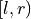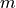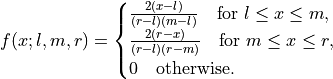# aesara.tensor.random.triangular#

aesara.tensor.random.triangular = <aesara.tensor.random.basic.TriangularRV object>[source]#

A triangular continuous random variable.

The probability density function for triangular within the intervaland mode(where the peak of the distribution occurs) is: